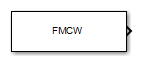# FMCW Waveform

Frequency-modulated continuous (FMCW) waveform source

## Library

Waveforms

`phasedwavlib`

•## Description

The FMCW Waveform block generates a frequency modulated continuous wave (FMCW) waveform with a specified sweep time and sweep bandwidth. The block output can be either an integer number of pulses or samples.

## Parameters

Sample rate

Specify the sample rate of the signal as a positive scalar. Units are hertz. The product of Sample rate and Sweep time must be integers.

Sweep time

Specify the duration, in seconds, of the upsweep or the downsweep of the signal as a scalar or row vector of positive, real numbers. The product of the Sample rate value and each Sweep time entry must be an integer.

To implement a varying sweep time, specify Sweep time as a row vector. The waveform uses successive entries of the vector as the sweep time for successive periods of the waveform. If the last element of the vector is reached, the process continues cyclically with the first entry of the vector.

If Sweep time and Sweep bandwidth are both row vectors, the vectors must have the same length.

If Sweep direction is `Up` or `Down`, the sweep period equals the sweep time. If Sweep direction is `Triangle`, the sweep period is twice the sweep time because each period consists of an upsweep segment and a downsweep segment.

Sweep bandwidth

Specify the bandwidth of the linear FM sweeping, in hertz, as a scalar or row vector of positive, real numbers.

To implement a varying bandwidth, specify Sweep bandwidth as a row vector. The waveform uses successive entries of the vector as the sweep bandwidth for successive periods of the waveform. If the waveform reaches the last element of the Sweep bandwidth vector, the process continues cyclically with the first entry of the vector.

If Sweep time and Sweep bandwidth are both row vectors, the vectors must have the same length.

Sweep direction

Specify the direction of the linear FM sweep as one of `Up`, `Down`, or `Triangle`.

Sweep interval

If you set this parameter value to `Positive`, the waveform sweeps in the interval between 0 and B, where B is the value of the Sweep bandwidth parameter. If you set this parameter to `Symmetric`, the waveform sweeps in the interval between –B/2 and B/2.

Output signal format

Specify the format of the output signal as `Sweeps` or `Samples`.

If you set this parameter to `Sweeps`, the output of the block is in the form of multiple sweeps. The number of sweeps is the value of the Number of sweeps in output parameter.

If you set this parameter to `Samples`, the output of the block is in the form of multiple samples. The number of samples is the value of the Number of samples in output parameter.

If the Sweep direction parameter is set to `Triangle`, each sweep is one-half of a period.

Number of sweeps in output

Specify the number of sweeps in the block output as a positive integer. This parameter appears only when you set Output signal format to `Sweeps`.

Number of samples in output

Number of samples in the block output, specified as a positive integer. This parameter appears only when you set Output signal format to `Samples`.

Simulate using

Block simulation method, specified as `Interpreted Execution` or ```Code Generation```. If you want your block to use the MATLAB® interpreter, choose `Interpreted Execution`. If you want your block to run as compiled code, choose `Code Generation`. Compiled code requires time to compile but usually runs faster.

Interpreted execution is useful when you are developing and tuning a model. The block runs the underlying System object™ in MATLAB. You can change and execute your model quickly. When you are satisfied with your results, you can then run the block using ```Code Generation```. Long simulations run faster than they would in interpreted execution. You can run repeated executions without recompiling. However, if you change any block parameters, then the block automatically recompiles before execution.

When setting this parameter, you must take into account the overall model simulation mode. The table shows how the Simulate using parameter interacts with the overall simulation mode.

When the Simulink® model is in `Accelerator` mode, the block mode specified using Simulate using overrides the simulation mode.

Acceleration Modes

 Block Simulation Simulation Behavior `Normal` `Accelerator` `Rapid Accelerator` `Interpreted Execution` The block executes using the MATLAB interpreter. The block executes using the MATLAB interpreter. Creates a standalone executable from the model. `Code Generation` The block is compiled. All blocks in the model are compiled.

## Ports

Note

The block input and output ports correspond to the input and output parameters described in the `step` method of the underlying System object. See link at the bottom of this page.

PortSupported Data Types
`Out`Double-precision floating point